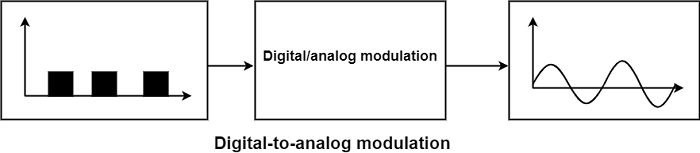# Explain Digital to Analog Conversion

Computer NetworkNetworkOperating System

#### Artificial Neural Network and Machine Learning using MATLAB

54 Lectures 4 hours

Digital-to-Analog conversion or digital-to-analog modulation is the phase of converting one of the features of an analog signal depending on the data in a digital signal (0s and 1s) when we communicate information from one system to another across a public access phone line.

For example, the initial data are digital, but mobile services use analog signals, the information should be transformed. The digital data should be inflected on an analog signal manipulated to a view like two definite values equivalent to binary 1 and binary 0.

The diagram demonstrates the relationship between the digital transformation, the digital-to-analog modulating hardware, and the effect analog signal.A sine wave is described by three features: amplitude, frequency and phase. When we alter one of these features, we generate a second form of that wave. Otherwise, we can say that the original wave describes binary 1, the variation can describe binary 0, and vice-versa.

Two primary issues must be defined in Digital to Analog Conversion.

• Bit Rate and Baud Rate
• Carrier Signals

Let us discuss these issues in greater detail −

## Bit Rate and Baud Rate

The two methods generally used in data communication are bit rate and baud rate. Bit rate is the number of bits that are sent during one second. Baud rate defines the number of signal units per second that are squared to represent those bits.

Bit rate equals the baud rate the number of bits represented by each signal unit. The baud rate equals the bit rate divided by the number of bits represented by each signal unit.

Example − The bit rate of a signal is 3000. If each signal element carries six bits, what is the baud rate?

Solution

Baud Rate = Bit Rate/Number of bits per signal element = $\frac{3000}{6}=500\:baud\:per\:second$.

## Carrier Signal

In analog transmission, the sending device makes a high-frequency signal that facilitates as a basis for the data signal. This base signal is known as the carrier signal or carrier frequency. The receiving device is accommodated to the frequency of the carrier signal that it anticipates from the sender. Digital information is modulated on the carrier signal by changing one or more of its characteristics (amplitude, frequency, and phase). This type of modification is known as modulation, and the data signal is known as the modulating signal.